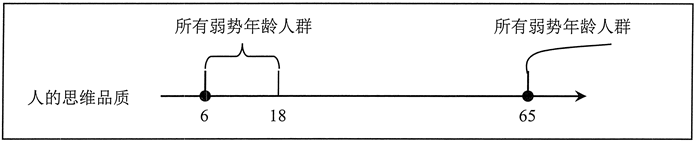• 内容
• 评论
• 相关

## 表达式的形式

```1  //数值直接量，计算后返回数值 1
"string"  //字符串直接量，计算后返回字符串“string”
false  //布尔直接量，计算后返回布尔值false
null  //特殊值直接量，计算后返回直接量null
/regexp/  //正则直接量，计算后返回正则表达式对象
{a : 1,b : "1"}  //对象直接量，计算后返回对象
[1,"1"]  //数组直接量，计算后返回数组
function(a,b){return a+b}  //函数直接量，计算后返回函数
a  //变量，计算后返回变量的值```

## 表达式的类型

1) 定义表达式，如定义变量、定义函数。

```var a = [];
var f = function(){};```

2) 初始化表达式，与定义表达式和赋值表达式常常混用。

```var a = [1,2];
var o = {x :1,y : 2};```

3) 访问表达式

```console.log([1,2] );  //返回2
console.log(({x : 1,y : 2}).x );  //返回1
console.log(({x : 1,y : 2}) ["x"]);  //返回1```

4) 调用表达式

```console.log(function(){return 1;}());  //返回1
console.log([1,3,2].sort());  //返回1,2,3```

5) 实例化对象表达式

```console.log(new Object());  //返回实例对象
console.log(new Object);  //返回实例对象```

## 表达式的运算顺序

#### 示例1

`(3-2-1) * (1+2+3) / (2*3*4);`

JavaScript 首先计算“3-2-1”子表达式，然后计算“1+2+3”子表达式，接着计算“2*3*4”子表达式，最后再执行乘法运算和除法运算。其逻辑顺序如下：

```var a = 1+2+3;
b = 2*3*4;
c = 3-2-1;
d = c * a / b;```

#### 示例2

`(a + b > c && a -b < c || a > b > c)`

`((a + b > c) && ((a - b < c) || (a >b >c)))`

## 表达式的优化

• 运算顺序分组优化。
• 逻辑运算结构优化。

#### 示例1

`if(age >= 6 && age < 18){ }`

`if(age >= 6 && age < 18 || age >= 65){ }`

`if((age >= 6 && age <18) || age >= 65){ }`0条评论